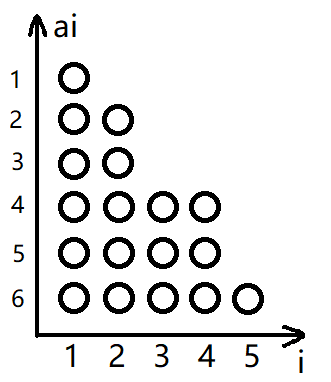# AGC 002E.Candy Piles(博弈论)

### $$Description$$

$$n\leq10^5,\ a_i\leq10^9$$

### $$Solution$$//8ms	896KB
#include <cstdio>
#include <cctype>
#include <algorithm>
#include <functional>
#define gc() getchar()
#define MAXIN 300000
typedef long long LL;
const int N=1e5+5;

int A[N];
char IN[MAXIN],*SS=IN,*TT=IN;

{
int now=0;register char c=gc();
for(;!isdigit(c);c=gc());
for(;isdigit(c);now=now*10+c-48,c=gc());
return now;
}

int main()
{
std::sort(A+1,A+1+n,std::greater<int>());
for(int i=1; i<=n; ++i)
if(i+1>A[i+1])
{
int ans=0;
for(int j=i+1; A[j]==i; ++j) ans^=1;
ans|=A[i]-i&1;
puts(ans?"First":"Second");
return 0;
}

return 0;
}

posted @ 2019-03-04 21:25  SovietPower  阅读(333)  评论(0编辑  收藏  举报